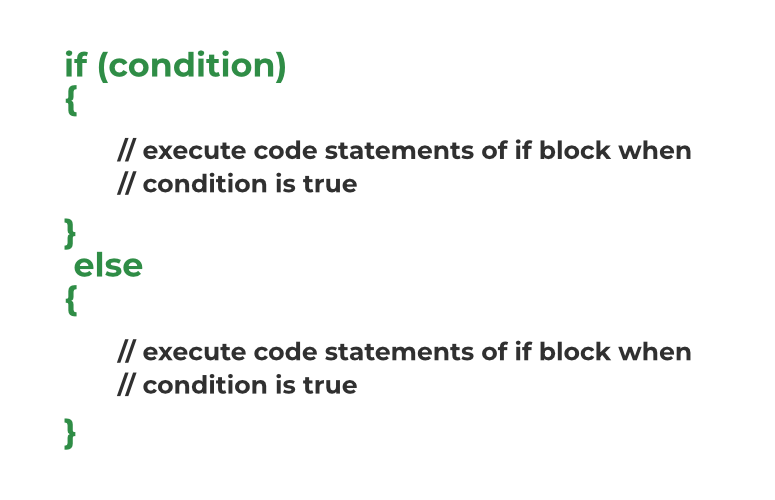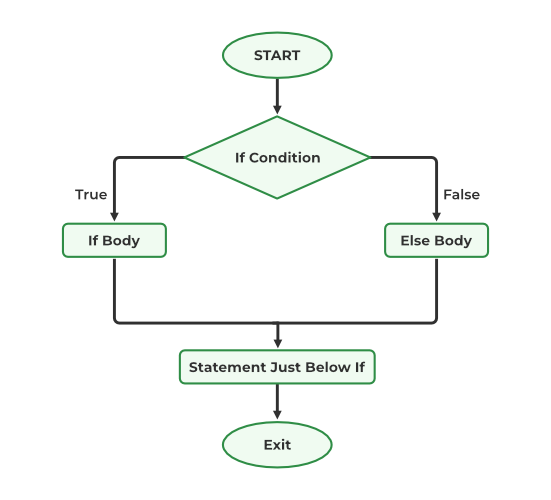# C if…else Statement

The if-else statement in C is a flow control statement used for decision-making in the C program. It is one of the core concepts of C programming. It is an extension of the if in C that includes an else block along with the already existing if block.

## C if Statement

The if statement in C is used to execute a block of code based on a specified condition.

The syntax of the if statement in C is:

```if (condition) {
// code to be executed if the condition is true
}```

## C if-else Statement

The if-else statement is a decision-making statement that is used to decide whether the part of the code will be executed or not based on the specified condition (test expression). If the given condition is true, then the code inside the if block is executed, otherwise the code inside the else block is executed.

## Syntax of if-else

```if (condition) {
// code executed when the condition is true
}
else {
// code executed when the condition is false
}```

## How to use if-else in C?

The following program demonstrates how to use if-else in C:

## C

 `// C Program to demonstrate the use of if-else statement` `#include `   `int` `main()` `{`   `    ``// if block with condition at the start` `    ``if` `(5 < 10) {`   `        ``// will be executed if the condition is true` `        ``printf``(``"5 is less than 10."``);` `    ``}`   `    ``// else block after the if block` `    ``else` `{`   `        ``// will be executed if the condition is false` `        ``printf``(``"5 is greater that 10."``);` `    ``}`   `    ``return` `0;` `}`

Output

`5 is less than 10.`

Note: Any non-zero and non-null values are assumed to be true, and zero or null values are assumed to be false.

## How if-else Statement works?

Working of the if-else statement in C is explained below:

1. When the program control first comes to the if-else block, the test condition is checked.
2. If the test condition is true:
• The if block is executed.
3. If the test condition is false:
• The else block is executed
4. After that, the program control continues to the statements below the if-else statement.Structure of if-else Syntax in C

We can understand the working of the if-else statement in C with the help of the flowchart.

## Flowchart of the if-else statementFlowchart of if-else in C

## Examples of if-else Statement in C

The following are two basic examples of the if-else statement that shows the use of the if-else statement in a C program.

### Example 1: C Program to check whether a given number is even or odd

For a given number to be even, it should be perfectly divisible by 2. We will use the if-else statement to check for this condition and execute different statements for when it is true and when it is false.

## C

 `// C Program to Demonstrate the working of if-else statement` `#include `   `int` `main()` `{`   `    ``// Some random number` `    ``int` `num = 9911234;`   `    ``// checking the condition at the start of if block` `    ``if` `(num % 2 == 0) {` `        ``// executed when the number is even` `        ``printf``(``"Number is even"``);` `    ``}` `    ``// else block` `    ``else` `{` `        ``// executed when the number is odd` `        ``printf``(``"Number is Odd"``);` `    ``}`   `    ``return` `0;` `}`

Output

`Number is even`

### Example 2. C Program to check whether a person is eligible to vote or not.

We know that a person is eligible to vote after he/she is at least 18 years old. Now we use this condition in the if-else statement to check the eligibility of the person.

## C

 `// C Program to check whether the person is eligible to vote` `// or not` `#include `   `int` `main()` `{`   `    ``// declaring age of two person` `    ``int` `p1_age = 15;` `    ``int` `p2_age = 25;`   `    ``// checking eligibility of person 1` `    ``if` `(p1_age < 18)` `        ``printf``(``"Person 1 is not eligible to vote.\n"``);` `    ``else` `        ``printf``(``"Person 1 is eligible to vote.\n"``);`   `    ``// checking eligiblity of person 2` `    ``if` `(p2_age < 18)` `        ``printf``(``"Person 2 is not eligible to vote.\n"``);` `    ``else` `        ``printf``(``"Person 2 is eligible to vote."``);`   `    ``return` `0;` `}`

Output

```Person 1 is not eligible to vote.
Person 2 is eligible to vote.```

You may notice that in the second example, we did not enclose the body of the if and else statement in the braces and still the code is running without error. This is because the C language allows the skipping of the braces around the body of the if-else statement when there is only one statement in the body.

• The if-else statement enables the user to execute different statements based on different conditions.
• It can evaluate test expressions of type int, char, boolean, and more.
• It helps in modifying the flow of the program.
• It is simple, efficient, and easier to read when there is less number of conditions.

• If there are a lot of if statements present, the code becomes unreadable and complex.
• It also becomes slower compared to the switch statement.

## Conclusion

In this article, we discussed how to use the if-else statement in C for making decisions in our program based on the specified conditions. Being the core concept of C programming, it is frequently used in almost all C programs.

## FAQs on if-else Statement in C

### 1. Can we skip braces around the body of the if-else block in C?

We can skip the braces of the body of the if or else block as long as there is only a single statement inside their body. We will get an error if there is more than one statement in the body without braces.

### 2. What is an if-else statement example?

Following is a simple example of the if-else statement in C:

## C

 `// C program to illustrate the use of if-else` `#include `   `int` `main()` `{`   `    ``// if else statement for true condition` `    ``if` `(1) {` `        ``printf``(``"The if block  is executed.\n"``);` `    ``}` `    ``else` `{` `        ``printf``(``"The else block is executed\n"``);` `    ``}`   `    ``return` `0;` `}`

Output

`The if block  is executed.`

### 3. What are the types of if-else statements in C?

There are 3 types of if-else statements in C which are as follows:

1. if Statement
2. if-else Statement

### 4. What is the syntax of the if-else statement?

The syntax of the if-else statement is:

```if (test expression) {
// if body
}
else {
// else body
}```

### Related Articles:

Whether you're preparing for your first job interview or aiming to upskill in this ever-evolving tech landscape, GeeksforGeeks Courses are your key to success. We provide top-quality content at affordable prices, all geared towards accelerating your growth in a time-bound manner. Join the millions we've already empowered, and we're here to do the same for you. Don't miss out - check it out now!

Previous
Next